AnyLogic

# Fluid Library statistics

At the end of the model execution the Fluid Library blocks dump their statistics to the DB logs.

There are four DB logs: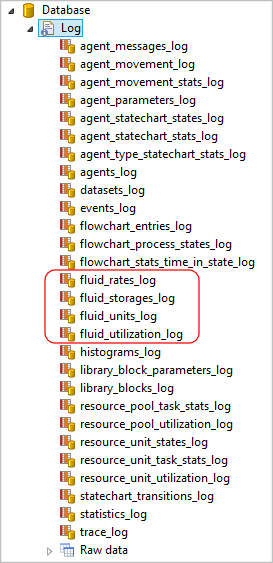DB views to collect statistics from:

1. The fluid_storages_log table looks like this: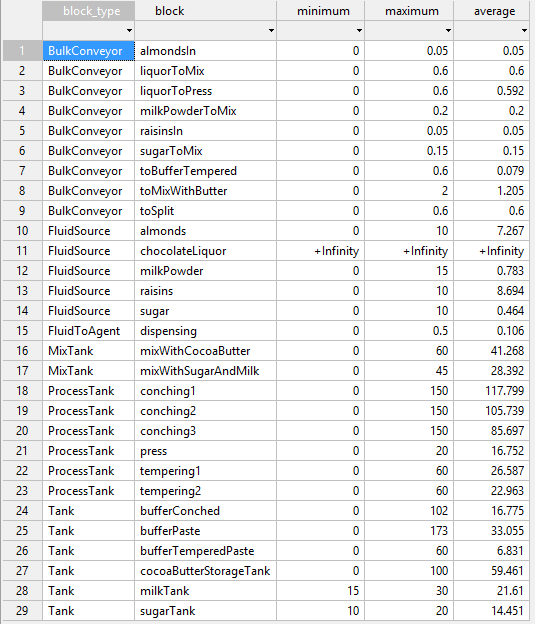Here every Fluid Library block that can hold non-zero amount of fluid saves historical minimum and maximum amount it ever contained, and time-average of the amount throughout the total operating time. Namely, the following blocks write to the table:
• Tank
• BulkConveyor
• FluidSource
• AgentToFluid
• FluidToAgent
• FluidPickup
• FluidDropoff
• MixTank
• ProcessTank
The FluidSource block would record infinity in case it worked in the Infinite capacity mode.
The Pipeline block does not dump its statistics as, once it gets full, it stays full forever, so the statistics is not meaningful.
The numbers in the table are in the default units (kilograms or cubic meters). To obtain the numbers in the units used by the user, you should look up the units in the fluid_units table and perform conversion.
2. Here is the fluid_rates_log table: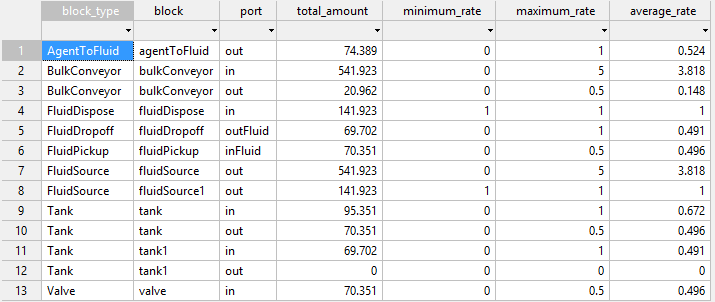The table contains the total amount of fluid that has passed through a port of a block, the historical minimum and maximum, and the time-average of the flow rate through the port. These blocks write to the table:
Again, the numbers in the table are in the default rate units (kilograms or cubic meters for the amounts and kilograms / second or cubic meters / second for the rates). To obtain the numbers in the units used by the user, you should look up the units in the fluid_units_log table and perform conversion.
3. The third table with statistical data is fluid_utilization_log.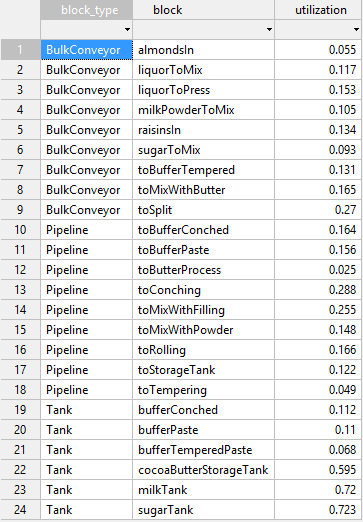The meaning of utilization is different for different blocks and means exactly the same as the function utilizatonTotal() of the block. Namely:
• Tank — the tank average utilization throughout the total operating time, i.e. the average amount divided by the tank capacity. If time has ran to infinity, the instant utilization is used.
• Pipeline — the average utilization of the pipeline bandwidth throughout the total operating time, i.e., the actual amount passed through the input divided by the maximum amount that could pass through, given the (possibly changing) maximum rate. If time has ran to infinity, the current utilization is returned. If the maximum rate has been set to unlimited even once, zero is assumed.
• BulkConveyor — the average utilization of the conveyor throughout the total operating time, i.e. the total amount of material that actually passed through the conveyor input divided by the maximum total amount that could pass through, given the (possibly changing) maximum input rate. If time has ran to infinity, the instant utilization is used.
• Valve — the average utilization of the valve bandwidth throughout the total operating time, i.e. the total amount of fluid that actually passed through the valve divided by the maximum total amount that could pass through, given the (possibly changing) open rate and closing times. If time has ran to infinity, the instant utilization is used. If the open rate has been set to unlimited even once, zero is assumed.
4. Finally, the table fluid_units_log contains the units the user used in the model to specify the block parameters: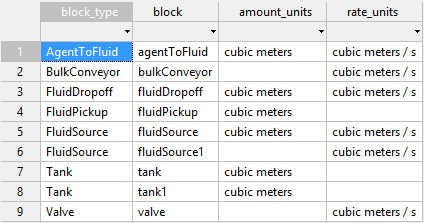If the user did not specify units (for example, the amount units in the FluidSource are not required if the block works in Infinite capacity mode), the table cell is empty.
The data in the tables fluid_storages_log and fluid_rates_log are NOT in the user-defined units, but in the default internal units (kilograms or cubic meters for the amounts and kilograms/second or cubic meters/second for the rates), so you may use this table to convert that data into a more user-friendly form.